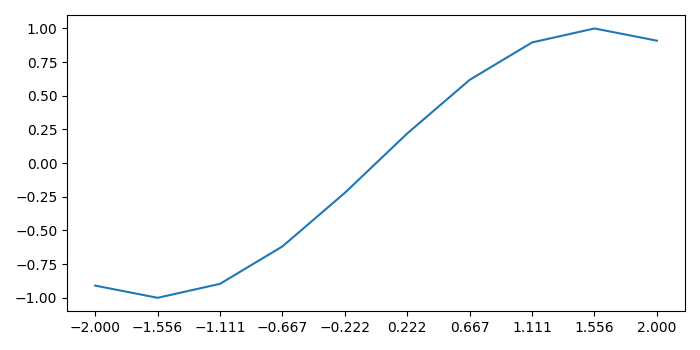# How to decrease the density of tick labels in subplots in Matplotlib?

MatplotlibPythonData Visualization

#### Python Data Science basics with Numpy, Pandas and Matplotlib

Most Popular

63 Lectures 6 hours

#### Data Visualization using MatPlotLib & Seaborn

11 Lectures 4 hours

#### MatPlotLib with Python

9 Lectures 2.5 hours

To decrease the density of tick labels in subplots in matplotlib, we can assign the minimum value to density.

## Steps

• Initialize a variable, density.

• Create x and y data points using numpy.

• Plot x and y data points using plot() method.

• Get or set the current tick locations and labels of the X-axis using xticks() method.

• To display the figure, use show() method.

## Example

import numpy as np
from matplotlib import pyplot as plt
plt.rcParams["figure.figsize"] = [7.00, 3.50]
plt.rcParams["figure.autolayout"] = True
density = 10
x = np.linspace(-2, 2, density)
y = np.sin(x)
plt.plot(x, y)
plt.xticks(x)
plt.show()

## Output